Courses

Test: Shapes (Geometry) - 3

20 Questions MCQ Test Mathematics for Class 3: NCERT | Test: Shapes (Geometry) - 3

Description
Attempt Test: Shapes (Geometry) - 3 | 20 questions in 40 minutes | Mock test for Class 3 preparation | Free important questions MCQ to study Mathematics for Class 3: NCERT for Class 3 Exam | Download free PDF with solutions
QUESTION: 1

Solution:
QUESTION: 2

Solution:
QUESTION: 3

Directions: In each of the following questions, identify the figure whose name and labelling is correct?

Solution:
QUESTION: 4

Directions: In each of the following questions, identify the figure whose name and labelling is correct?

Solution:
QUESTION: 5

Which among the following is correct information for the plane shapes

Solution:
QUESTION: 6

A point has _________.

Solution:
QUESTION: 7

A line is made up of many points placed ___________.

Solution:
QUESTION: 8

Which among the following is an example of horizontal line?

Solution:
QUESTION: 9

Which among the following is an example of vertical line?

Solution:
QUESTION: 10

Which among the following is an example of parallel lines?

Solution:
QUESTION: 11

Identify the slanting lines in the given figures.

Solution:
QUESTION: 12

Identify the figure which has 4 sides.

Solution:
QUESTION: 13

Directions: Consider the following and answer the questions given below: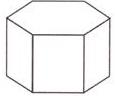The number of faces in the figure, is __________.

Solution:
QUESTION: 14

Directions: Consider the following and answer the questions given below: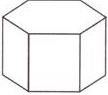The number of edges(s) in the figure is __________.

Solution:
QUESTION: 15

Directions: Consider the following and answer the questions given below: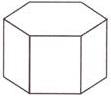The number of vertices in the figure is _____

Solution:
QUESTION: 16

Which of the following solids has no edges?

Solution:

sphere has no edges and therefore no corners. It has one curved face that goes all the way around. A square based pyramid, a triangular based pyramid (prism) and a cone have a point at the top. A cuboid and cube have four edges.

QUESTION: 17

Count the number of cubes in the figure given below: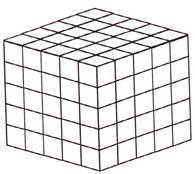Solution:
QUESTION: 18

Directions: Consider the figure given below: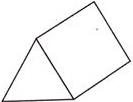Based on the figure, answer the following questions:

Q. The number of vertices in the figure is _________

Solution:
QUESTION: 19

Directions: Consider the figure given below: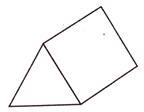Based on the figure, answer the following questions:

Q. The number of edges in the figure is _________

Solution:

The number of edges in the figure is 9.

QUESTION: 20

Directions: Consider the figure given below:Based on the figure, answer the following questions:

Q. The number of faces in the figure is ________

Solution:Use Code STAYHOME200 and get INR 200 additional OFF Use Coupon Code

Track your progress, build streaks, highlight & save important lessons and more!

Similar ContentRelated tests